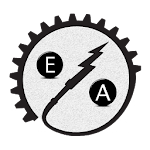ID: com.aliens_studio.EMAssetTrial1

• Author:

• Version:

Varies with device

• Update on:

## The description of ElectroMechanical Calculation

E.M Asset (Electro Mechanical assistant)
1- CO2 Calculation
Design firefighting systems with carbon dioxide get: -
Total CO2 Quantity.
Cylinder Size Required.
Number of CO2 Cylinder Required.
Number of Nozzles.
2- FM-200 Fire Suppression System
Calculate FM-200 Total flooding Quantity.
3- Sprinkler system calculation
Choose the hazard Classification (light , ordinary or extra ) and Get the number of sprinkler in designed area, space between Sprinkler in length and in width.
4- Fire Alarm Detectors number.
Get the number of smoke fire alarm detector based on number of air change and maximum space between detectors.
Get the number of heat fire alarm detector based on height of the designed area and maximum space between detectors.
5- Power Calculations
a- Get Current
Based on power and power factor and operating voltage get: -
Circuit breaker current (I Circuit breaker).
Cable Current (I Cable).
b- Voltage drop Calculate: -
Voltage drop.
Voltage drop percentage.
Wire resistance.
c- Short Circuits: -
Three-Phase Short Circuits
Single-Phase Short Circuits Line-to-Line (L-L) Fault and Line-to-Neutral (L-N) Fault
Calculate: -
Is.c. sym RMS (short circuit symmetrical RMS current).
Is.c. (motor short circuit contribution).
I total s.c. (Total Short current).
d- Earth System Calculation: -
Total Resistance of Earthing System.
Conductor network resistance.
Multiple electrode resistance.
electrode resistance.
6- Lighting Calculations: -
a- Lumens to lux calculator.
b- Lux to lumens calculator.
c- Recommended Light Levels.
d- Calculate number of luminaires in specific area.
7- Unit Converter: -
Length units (millimeter, centimeter, meter, inch, foot, Yard, mile and Kilometer).
Area units (millimeter square…………..., Yard square, mile square etc.)
Volume units (millimeter cubic…………..., Yard cubic, mile cubic etc.).
Mass (Gram, kilogram, tonne , Ton(Uk) , Ton (Us) , pound , Ounce).
Temperature (Celsius, Fahrenheit, Kelvin, Rnkine and Reaumur).
Pressure (Joule, kilojoule, Cal, kiloCal and KWh).
Power (Watt, kilowatt and Horsepower).